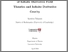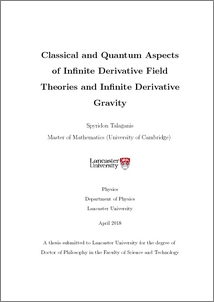# Classical and quantum aspects of infinite derivative field theories and infinite derivative gravity

Talaganis, Spyridon (2018) Classical and quantum aspects of infinite derivative field theories and infinite derivative gravity. PhD thesis, UNSPECIFIED.Preview
PDF (2018talaganisphd)
2018talaganisphd.pdf - Published Version

## Abstract

The objective of this thesis is to study classical and quantum aspects of infinite derivative field theories and infinite derivative gravity. In- finite derivative theories of gravity can be made free from ghosts and classical singularities. In order to avoid ghosts, one modifies the graviton propagator by employing entire functions so that no new poles are introduced apart from the pole corresponding to the massless graviton of General Relativity. Inspired by infinite derivative gravity, we consider an infinite derivative scalar toy model and demonstrate renormalisability when the loop-order is arbitrarily large. Moreover, scattering diagrams within the framework of infinite derivative field theories are explicitly evaluated and it is shown that the cross section can be made finite. Finally, we perform a Hamiltonian analysis of an infinite derivative gravitational theory with a simpler action containing only the Ricci scalar and compute the number of relevant degrees of freedom.

Item Type:
Thesis (PhD)
Departments:
ID Code:
88133
Deposited By:
Deposited On:
06 Oct 2017 20:18
Refereed?:
No
Published?:
Unpublished